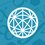# Minimum Distance Between 2 CurvesI was trying ( unsuccessfully ) to find the minimum distance between two parabolas and thought that I could do that by finding the minimum distance between two parallel tangents to the two parabolas. However there comes a case when ( as shown in figure ) the tangents are indeed parallel and the distance between them is also minimum but ( as shown by the green line ) the actual distance between the point of contacts is not the distance between the parallel lines but much more ... How do I do such problems ??Note by Santanu Banerjee
6 years, 8 months ago

This discussion board is a place to discuss our Daily Challenges and the math and science related to those challenges. Explanations are more than just a solution — they should explain the steps and thinking strategies that you used to obtain the solution. Comments should further the discussion of math and science.

When posting on Brilliant:

• Use the emojis to react to an explanation, whether you're congratulating a job well done , or just really confused .
• Ask specific questions about the challenge or the steps in somebody's explanation. Well-posed questions can add a lot to the discussion, but posting "I don't understand!" doesn't help anyone.
• Try to contribute something new to the discussion, whether it is an extension, generalization or other idea related to the challenge.

MarkdownAppears as
*italics* or _italics_ italics
**bold** or __bold__ bold
- bulleted- list
• bulleted
• list
1. numbered2. list
1. numbered
2. list
Note: you must add a full line of space before and after lists for them to show up correctly
paragraph 1paragraph 2

paragraph 1

paragraph 2

[example link](https://brilliant.org)example link
> This is a quote
This is a quote
    # I indented these lines
# 4 spaces, and now they show
# up as a code block.

print "hello world"
# I indented these lines
# 4 spaces, and now they show
# up as a code block.

print "hello world"
MathAppears as
Remember to wrap math in $$ ... $$ or $ ... $ to ensure proper formatting.
2 \times 3 $2 \times 3$
2^{34} $2^{34}$
a_{i-1} $a_{i-1}$
\frac{2}{3} $\frac{2}{3}$
\sqrt{2} $\sqrt{2}$
\sum_{i=1}^3 $\sum_{i=1}^3$
\sin \theta $\sin \theta$
\boxed{123} $\boxed{123}$

Sort by:

Minimum distance will be along common normal. Use little calculus & co-ordinate geometry to get it.

- 6 years, 8 months ago

I don't think calculus will be needed....work using the parabola y^2=4ax....and use the parametric form of the equation i.e. (at^2,2at)...then write equation for both the normals and equate them....works beautifully!!!

- 6 years, 5 months ago

How can we equate them there would be two variable t1 and t2

- 3 years, 9 months ago

Ooh! My favorite! You have to set up a distance formula, with each equation as a point! It's awesome! From there, you simplify, and use basic algebra to minimize! Great post!

- 6 years, 5 months ago

so its like distance between (x,f(x)) and (y,g(y))

- 2 years, 3 months ago

can be solved by making the equation in a variable involving parametric equations for the two curves .. try to get it in one single parameter and then differentiate to get the critical point.. !!

- 6 years, 8 months ago

cool

- 5 years, 4 months ago

You can find the symmetry line.......as an example y=x is the line of symmetry between y^2=4x and x^2=4y double the dist from one parabola to y=x and you get the distance

- 5 years, 3 months ago

lagrange methode in calculus maybe help

- 6 years, 8 months ago